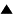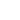# Entropy and the Laws of Thermodynamics

The principal energy laws that govern every organization are derived from two famous laws of thermodynamics. The second law, known as Carnot's principle, is controlled by the concept of entropy.

Today the word entropy is as much a part of the language of the physical sciences as it is of the human sciences. Unfortunately, physicists, engineers, and sociologists use indiscriminately a number of terms that they take to be synonymous with entropy, such as disorder, probability, noise, random mixture, heat; or they use terms they consider synonymous with antientropy, such as information, neguentropy, complexity, organization, order, improbability.

There are at least three ways of defining entropy:

• in terms of thermodynamics (the science of heat), where the names of Mayer, Joule, Carnot, and Clausius (1865) are important;
• in terms of statistical theory, which fosters the equivalence of entropy and disorder -- as a result of the work of Maxwell, Gibbs, and Boltzmann (1875), and
• in terms of information theory, which demonstrates the equivalence of neguentropy (the opposite of entropy) and information -- as a result of the work of Szilard, Gabor, Rothstein, and Brillouin (1940-1950)
The two principal laws of thermodynamics apply only to closed systems, that is, entities with which there can be no exchange of energy, information, or material. The universe in its totality might be considered a closed system of this type; this would allow the two laws to be applied to it.

The first law of thermodynamics says that the total quantity of energy in the universe remains constant. This is the principle of the conservation of energy. The second law of thermodynamics states that the quality of this energy is degraded irreversibly. This is the principle of the degradation of energy.

The first principle establishes the equivalence of the different forms of energy (radiant, chemical, physical, electrical, and thermal), the possibility of transformation from one form to another, and the laws that govern these transformations. This first principle considers heat and energy as two magnitudes of the same physical nature

About 1850 the studies of Lord Kelvin, Carnot, and Clausius of the exchanges of energy in thermal machines revealed that there is a hierarchy among the various forms of energy and an imbalance in their transformations. This hierarchy and this imbalance are the basis of the formulation of the second principle.

In fact physical, chemical, and electrical energy can be completely changed into heat. But the reverse (heat into physical energy, for example) cannot be fully accomplished without outside help or without an inevitable loss of energy in the form of irretrievable heat. This does not mean that the energy is destroyed; it means that it becomes unavailable for producing work. The irreversible increase of this nondisposable energy in the universe is measured by the abstract dimension that Clausius in 1865 called entropy (from the Greek entrope, change).

The concept of entropy is particularly abstract and by the same token difficult to present. Yet some scientists consider it intuitively; they need only refer mentally to actual states such as disorder, waste, and the loss of time or information. But how can degraded energy, or its hierarchy, or the process of degradation be truly represented?

There seems to be a contradiction between the first and second principles. One says that heat and energy are two dimensions of the same nature; the other says they are not, since potential energy is degraded irreversibly to an inferior, less noble, lower-quality form--heat. Statistical theory provides the answer. Heat is energy; it is kinetic energy that results from the movement of molecules in a gas or the vibration of atoms in a solid. In the form of heat this energy is reduced to a state of maximum disorder in which each individual movement is neutralized by statistical laws.

Potential energy, then, is organized energy; heat is disorganized energy. And maximum disorder is entropy. The mass movement of molecules (in a gas, for example) will produce work (drive a piston). But where motion is ineffective on the spot and headed in all directions at the same time, energy will be present but ineffective. One might say that the sum of all the quantities of heat lost in the course of all the activities that have taken place in the universe measures the accumulation of entropy.

One can generalise further. Thanks to the mathematical relation between disorder and probability, it is possible to speak of evolution toward an increase in entropy by using one or the other of two statements: "left to itself, an isolated system tends toward a state of maximum disorder" or "left to itself, an isolated system tends toward a state of higher probability." (this is illustrated most simply by the example of the box with two compartments). These equivalent expressions can be summarized:

• Potential energy -> entropy
• Ordered energy -> disorganized energy (heat)
• High-quality energy -> heat (low-grade energy)
• Order -> disorder
• Improbability -> probability

The concepts of entropy and irreversibility, derived from the second principle, have had a tremendous impact on our view of the universe. In breaking the vicious circle of repetitiveness in which the ancients were trapped, and in being confronted with biological evolution generating order and organization, the concept of entropy indirectly opens the way to a philosophy of progress and development (see: the direction of evolution). At the same time it introduces the complementarity between the "two great drifts of the universe" described in the works of Bergson and Teilhard de Chardin.

The image of the inexorable death of the universe, as suggested by the second principle, has profoundly influenced our philosophy, our ethics, our vision of the world, and even our art. The thought that by the very nature of entropy the ultimate and only possible future for man is annihilation has infiltrated our culture like a paralysis. This consideration led Leon Brillouin to ask, "How is it possible to understand life when the entire world is ordered by a law such as the second principle of thermodynamics, which points to death and annihilation?"

 Author J.de Rosnay Date Jul 3, 1998
 HomeMetasystem Transition TheoryEvolutionary TheorySelf-organization Up Prev.Next Down